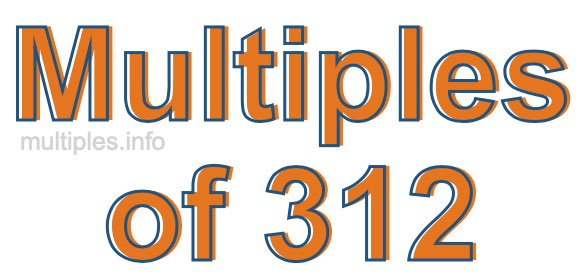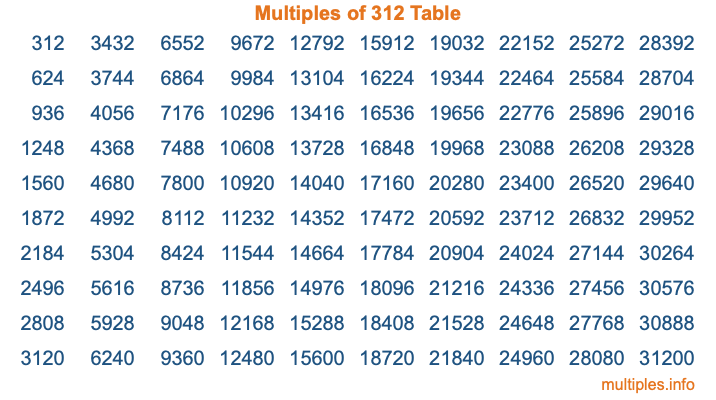Multiples of 312Welcome to the Multiples of 312 page. Here we will first teach you everything you will ever need to know about the multiples of 312, and then give you a study guide summary of everything we taught you to make sure you remember it all. Use this page to look up facts and learn information about the multiples of 312. This page will make you a multiples of three hundred twelve expert!

Definition of Multiples of 312
Multiples of 312 are all the numbers that when divided by 312 equal an integer. Each of the multiples of 312 are called a multiple. A multiple of 312 is created by multiplying 312 by an integer.

Therefore, to create a list of multiples of 312, you start with 1 multiplied by 312, then 2 multiplied by 312, then 3 multiplied by 312, and so on for as long as you want. Thus, the list of the first five multiples of 312 is 312, 624, 936, 1248, and 1560. To see a larger list of multiples of 312, see the printable image of Multiples of 312 further down on this page. We also have a category where you can choose any nth multiple of 312.

Multiples of 312 Checker
The Multiples of 312 Checker below checks to see if any number of your choice is a multiple of 312. In other words, it checks to see if there is any number (integer) that when multiplied by 312 will equal your number. To do that, we divide your number by 312. If the the quotient is an integer, then your number is a multiple of 312.

Is  a multiple of 312?

Least Common Multiple of 312 and ...
A Least Common Multiple (LCM) is the lowest multiple that two or more numbers have in common. This is also called the smallest common multiple or lowest common multiple and is useful to know when you are adding our subtracting fractions. Enter one or more numbers below (312 is already entered) to find the LCM.

Check out our LCM Calculator if you need more details about the Least Common Multiple or if you need the LCM for different numbers for adding and subtraction fractions.

nth Multiple of 312
As we stated above, 312 is the first multiple of 312, 624 is the second multiple of 312, 936 is the third multiple of 312, and so on. Enter a number below to find the nth multiple of 312.

th multiple of 312

Multiples of 312 vs Factors of 312
312 is a multiple of 312 and a factor of 312, but that is where the similarities end. All postive multiples of 312 are 312 or greater than 312. All positive factors of 312 are 312 or less than 312.

Below is the beginning list of multiples of 312 and the factors of 312 so you can compare:

Multiples of 312: 312, 624, 936, 1248, 1560, etc.

Factors of 312: 1, 2, 3, 4, 6, 8, 12, 13, 24, 26, 39, 52, 78, 104, 156, 312

As you can see, the multiples of 312 are all the numbers that you can divide by 312 to get a whole number. The factors of 312, on the other hand, are all the whole numbers that you can multiply by another whole number to get 312.

It's also interesting to note that if a number (x) is a factor of 312, then 312 will also be a multiple of that number (x).

Multiples of 312 vs Divisors of 312
The divisors of 312 are all the integers that 312 can be divided by evenly. Below is a list of the divisors of 312.

Divisors of 312: 1, 2, 3, 4, 6, 8, 12, 13, 24, 26, 39, 52, 78, 104, 156, 312

The interesting thing to note here is that if you take any multiple of 312 and divide it by a divisor of 312, you will see that the quotient is an integer.

Multiples of 312 Table
Below is an image of the first 100 multiples of 312 in a table. The table is in chronological order, column by column. The first column has the first ten multiples of 312, the second column has the next ten multiples of 312, and so on.The Multiples of 312 Table is also referred to as the 312 Times Table or Times Table of 312. You are welcome to print out our table for your studies.

Negative Multiples of 312
Although not often discussed or needed in math, it is worth mentioning that you can make a list of negative multiples of 312 by multiplying 312 by -1, then by -2, then by -3, and so on, to get the following list of negative multiples of 312:

-312, -624, -936, -1248, -1560, etc.

Multiples of 312 Summary
Below is a summary of important Multiples of 312 facts that we have discussed on this page. To retain the knowledge on this page, we recommend that you read through the summary and explain to yourself or a study partner why they hold true.

There are an infinite number of multiples of 312.

A multiple of 312 divided by 312 will equal a whole number.

312 divided by a factor of 312 equals a divisor of 312.

The nth multiple of 312 is n times 312.

The largest factor of 312 is equal to the first positive multiple of 312.

312 is a multiple of every factor of 312.

312 is a multiple of 312.

A multiple of 312 divided by a divisor of 312 equals an integer.

312 divided by a divisor of 312 equals a factor of 312.

Any integer times 312 will equal a multiple of 312.

Multiples of a Number
Here you can get the multiples of another number, all with the same attention to detail as we did for multiples of 312 on this page.

Multiples of
Multiples of 313
Did you find our page about multiples of three hundred twelve educational? Do you want more knowledge? Check out the multiples of the next number on our list!